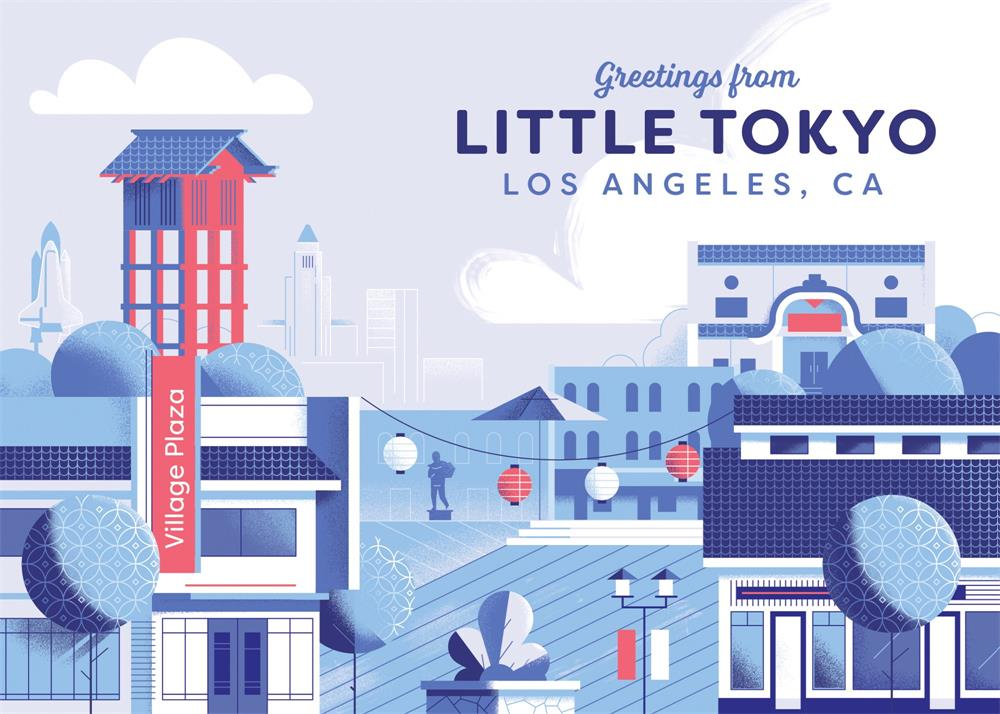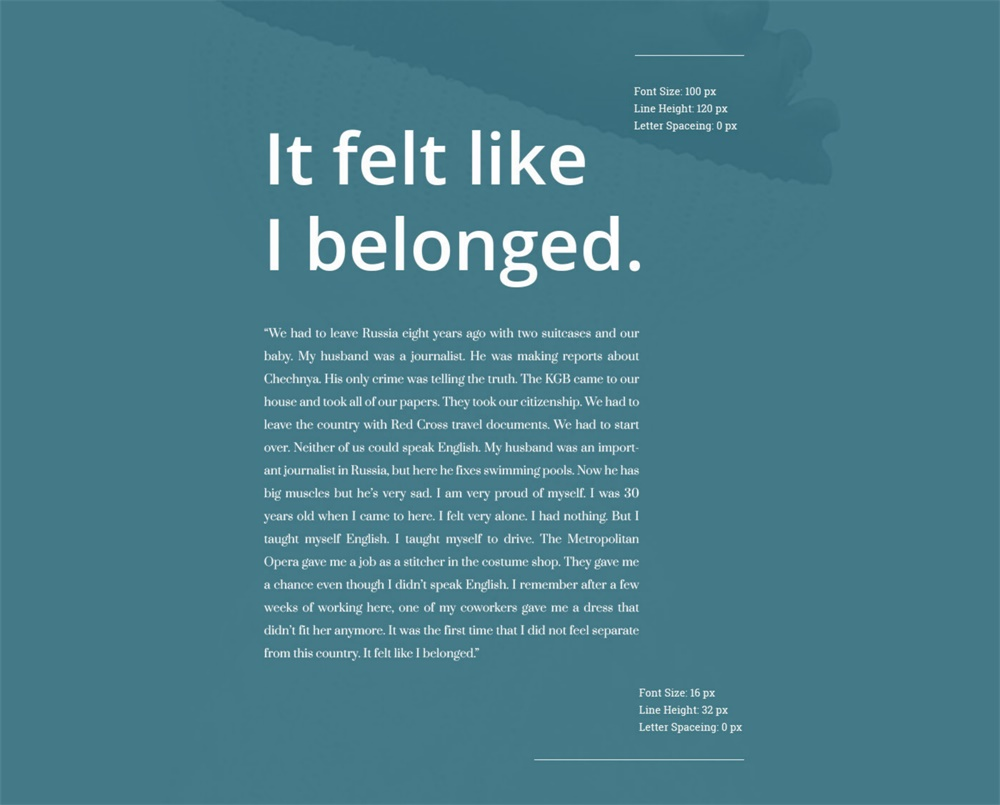一、确定目标△ 选择合适的插图风格字体二、确定受众△ Rosemary Sassoon开发了Sassoon Primary字体

三、寻找灵感

1. 字体灵感Typ.io 收录了大量已上线的字体案例资源

（译者注：亲自试验了，能很方便的查字体了，有个地方要注意的是，必须等网站加载完成了，点击那个插件图标才有效果哦。）

2. 搭配灵感△ 字体组合和字体本身一样重要

FontPair 还专门为谷歌字体设计了字体搭配灵感。你可以按字体类型组合进行排序，例如：无衬线字体和衬线字体或者衬线字体和衬线字体。四、选择字体△ 在选择字体之前，先研究一下产品目标△ 搭配对比更大的字体

五、确定字体大小Golden Ratio (1:1.618)1.000 x 1.618 = 1.6181.618 x 1.618 = 2.6182.618 x 1.618 = 4.2364.236 x 1.618 = 6.8546.854 x 1.618 = 11.089

Golden Ratio (1:1.618)…11.089 x 1.618 = 17.94217.942 x 1.618 = 29.0329.030 x 1.618 = 46.97146.971 x 1.618 = 75.99975.999 x 1.618 = 122.966

Minor Second 15:16 Major Second 8:9Minor Third 5:6Major Third 4:5…

Perfect Fourth (3:4)…9.969 x 1.333 = 13.28813.288 x 1.333 = 17.71317.713 x 1.333 = 23.61223.612 x 1.333 = 31.47531.475 x 1.333 = 41.95641.956 x 1.333 = 55.927

六、创建一套字体规范△ 在sketch中设置字体共享样式△ 使用规范来保证设计的一致性「一网打尽！快速上手字体设计」